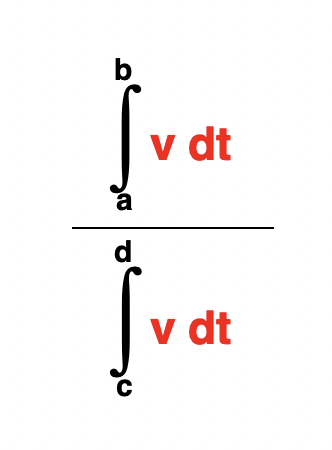# Integral with limits under #frac{...}{...}

#1

Hi,
I want to draw a fraction with an integral (with limits) in the numerator, but TLatex::DrawLatex complains about
*ERROR<TLatex>: Missing denominator for #frac

Here is the MWE:

void drawl()
{
TCanvas* c1 = new TCanvas();
TH1I* h = new TH1I("h", ";X;Y", 10, 0., 10.);
h->Draw();

TLatex* l = new TLatex();
//      l->DrawLatex(5, 0.5, "#frac{#int v dt}{c}");//this works
l->DrawLatex(5, 0.5, "#frac{#int^{a} v dt}{c}");//this does not work
}


The integral without limits works fine, but the integral with limits breaks something down. What’s the reason for this?

ROOT Version: 6.12/06
Platform: x86_64-slc6-gcc62-opt
Compiler: x86_64-slc6-gcc62-opt

#2

Yes it seems there is a problem… I am trying to find a work around

#3

One possibility is to use TMathText interpreter:

TLatex* l = new TLatex();
l->DrawLatex(.5, 0.5, "\\frac{\\int^{a} v dt}{c}");


#4

Yes, this works for something simple. What I really want to achieve in the end is to make this thing work:

l->DrawLatex(.5, .5, "#frac{#int^{b}_{a} #color{v dt}}{#int^{d}_{c} #color{v dt}}");


I’m particularly interested in this #color{...} construction - if I replace the # with the \\, there is no colorized output. If I don’t, the # is ignored and I see the word color in the numerator and denominator.

#5

I see. In that case the only way I can think of is to make the fraction with two TLatex.

{
TLatex *   tex = new TLatex(0.5,0.5,"#int^{b}_{a} #color{v dt}");
tex->Draw();
tex = new TLatex(0.5,0.3157895,"#int^{d}_{c} #color{v dt}");
tex->Draw();
TLine *line = new TLine(0.47,0.43,0.62,0.43);
line->Draw();
}#6

This topic was automatically closed 14 days after the last reply. New replies are no longer allowed.